Question

calculate reactions at each hinge

Consider the beam:

The beam has six supports(hinges), the distances are shown here.

Determine the values for each R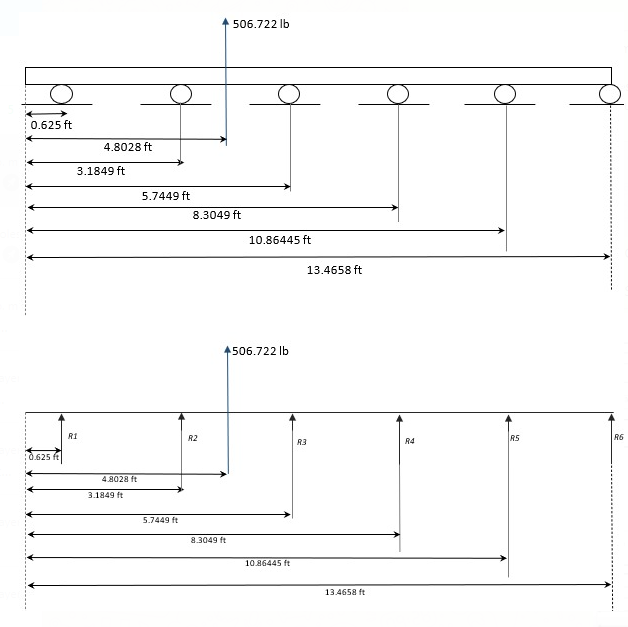> Please show all work and write neatly so I can understand how to do this on my own

Melissa Pineda Wed, Apr 21, 2021 8:43 AM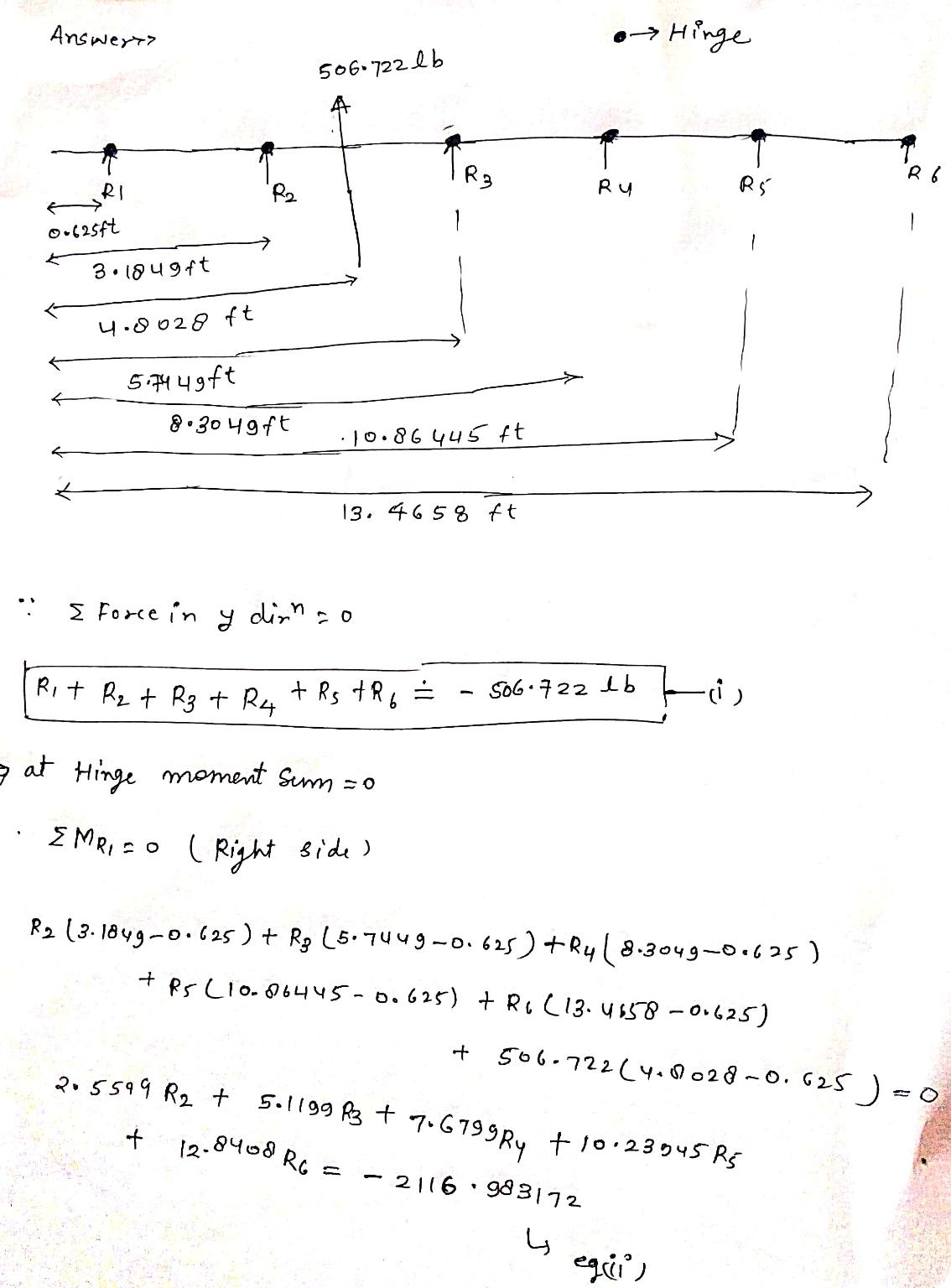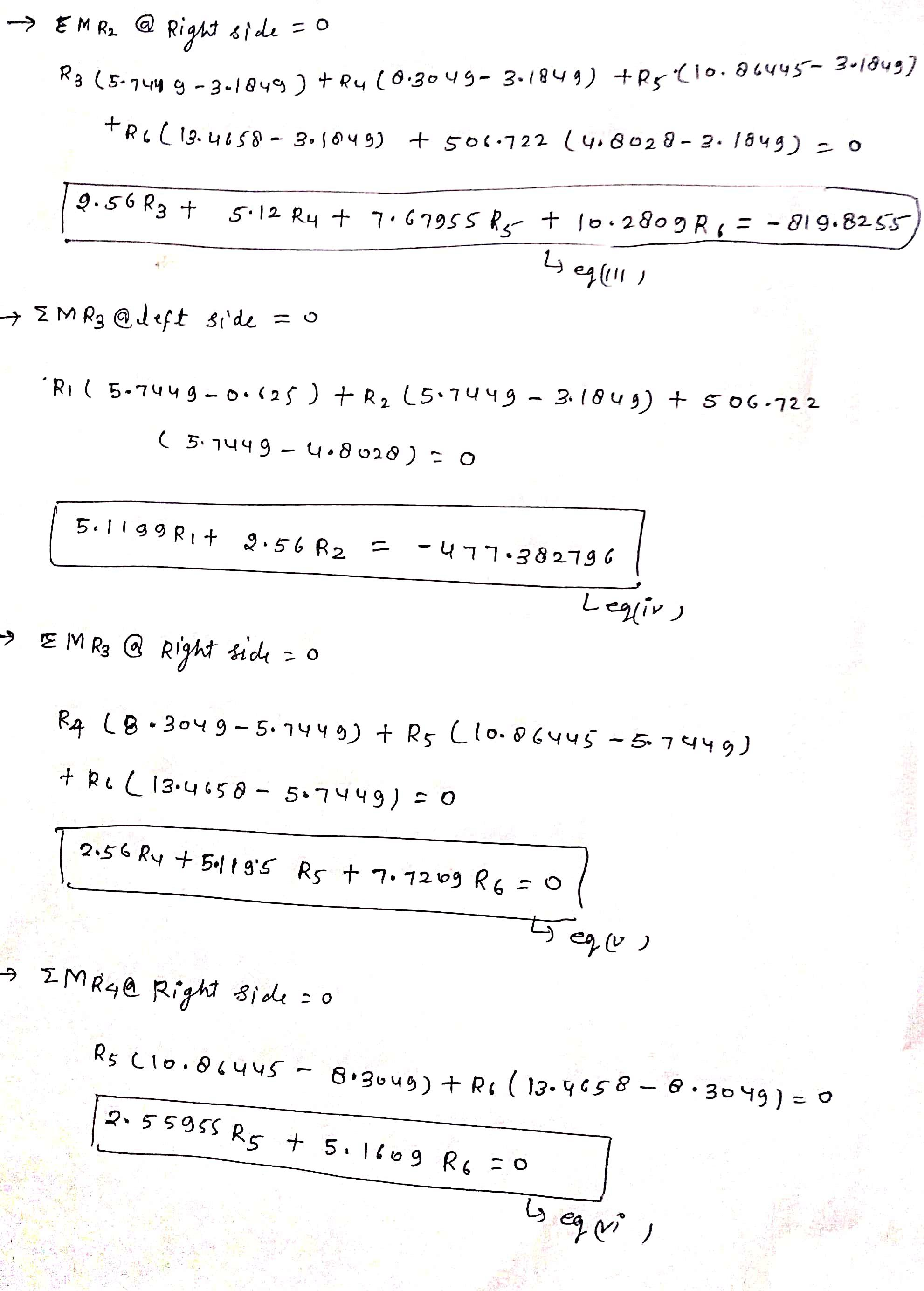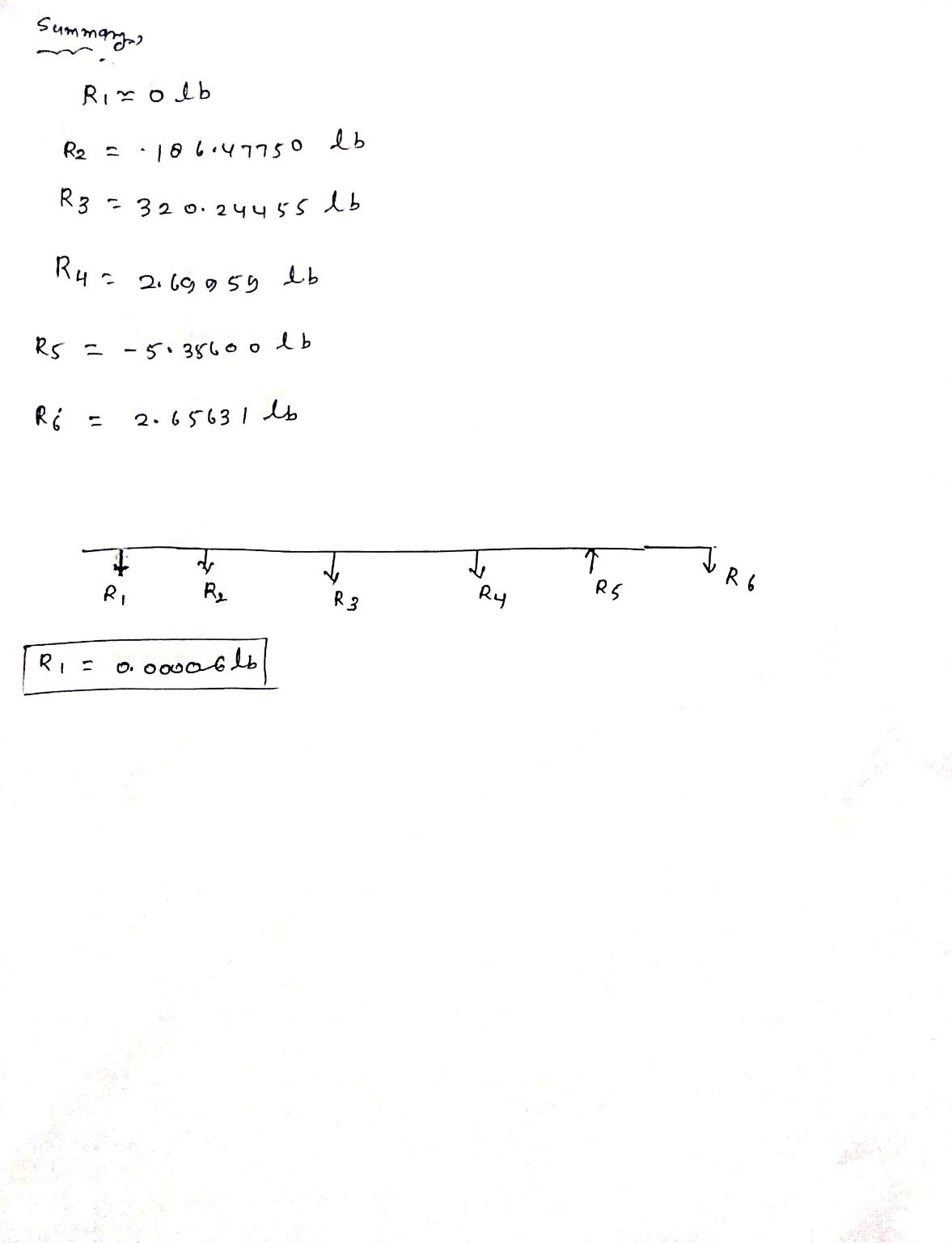Earn Coins

Coins can be redeemed for fabulous gifts.

Similar Homework Help Questions
• Determing the reactions at the supports

The compound beam is fixed at A and supported by a rocker at B and C. There are hinges (pins) at D and E. Determine the reactions at the supports.[from "Structural Analysis, 8th Edition" by R.C. Hibbeler]

• 1) Determine the reactions at the supports for the structures shown. The support at A is...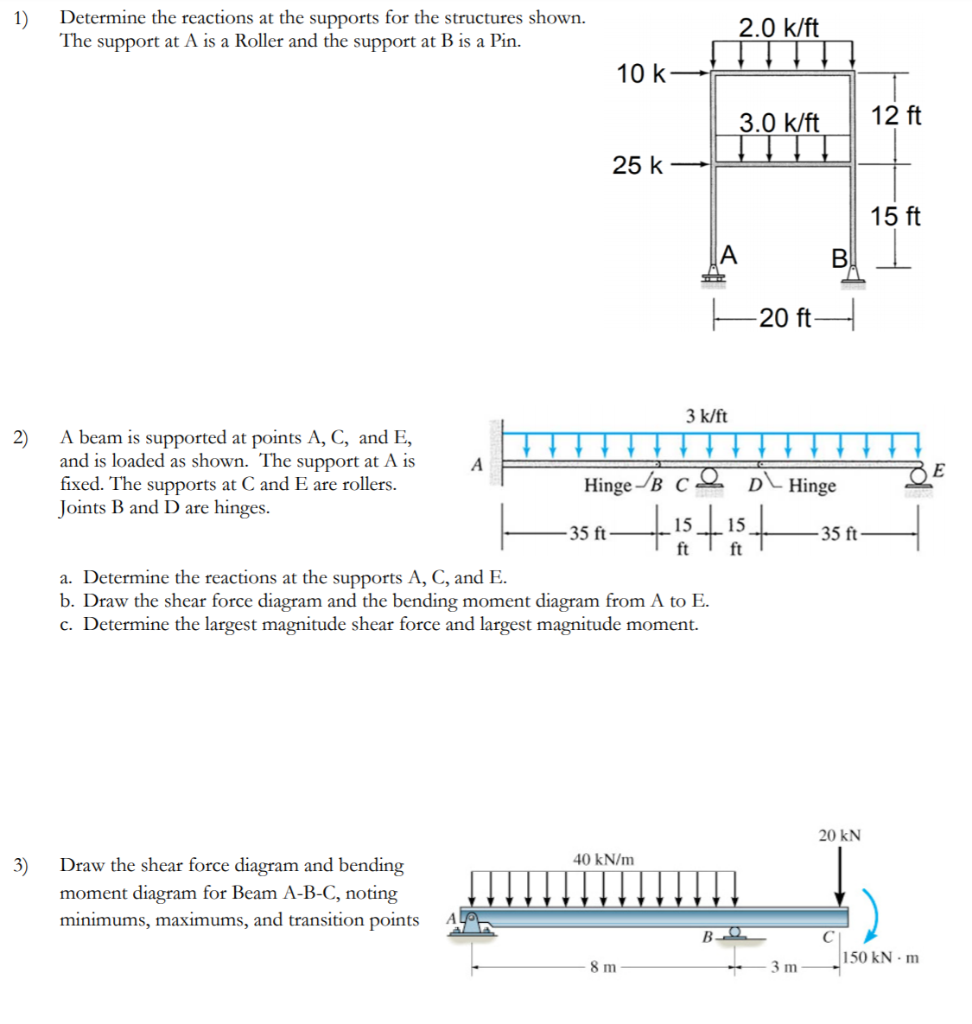1) Determine the reactions at the supports for the structures shown. The support at A is a Roller and the support at B is a Pin. 2.0 k/ft M 10 k- 3.0 k/ft 12 ft 25 k- 25k-Pokift 15 ft B! 1 F20ft- 2) 2 3 k/ft A beam is supported at points A, C, and E, and is loaded as shown. The support at A is fixed. The supports at C and E are rollers. Hinge-B ce Dl Hinge...

• The beam ABC is supported at A with a guided support (fixed with roller) and hinge...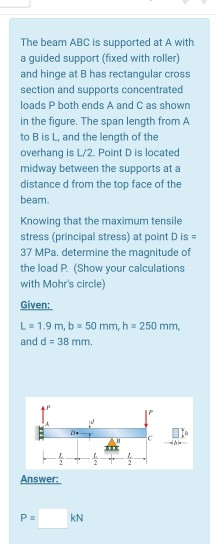The beam ABC is supported at A with a guided support (fixed with roller) and hinge at B has rectangular cross section and supports concentrated loads P both ends A and C as shown in the figure. The span length from A to Bis L, and the length of the overhang is 1/2. Point D is located midway between the supports at a distance d from the top face of the beam Knowing that the maximum tensile stress (principal stress)...

• Question 1 (a) Abeam has a fixed support at A and roller supports at D and E as shown in Fig. Q.la below. Internal...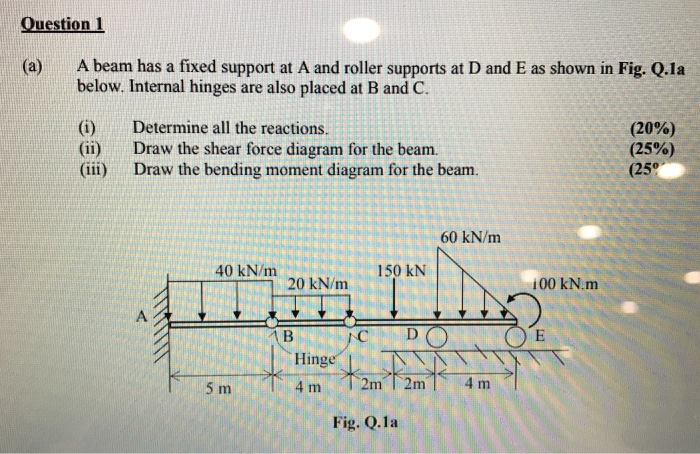Question 1 (a) Abeam has a fixed support at A and roller supports at D and E as shown in Fig. Q.la below. Internal hinges are also placed at B and C. (i) Determine all the reactions (ii) Draw the shear force diagram for the beam. (iii) Draw the bending moment diagram for the beam. (20%) (25%) (25° 60 kN/m 40 kN/m 20 kN/m 150 kN 100 kN.m Hinge Fig. Q.1a

• The beam ABC is supported at A with a guided support (fixed with roller) and hinge...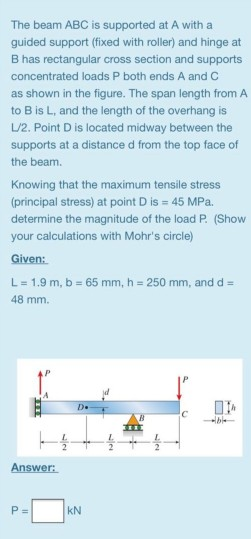The beam ABC is supported at A with a guided support (fixed with roller) and hinge at B has rectangular cross section and supports concentrated loads P both ends A and C as shown in the figure. The span length from A to B is L, and the length of the overhang is 1/2. Point D is located midway between the supports at a distance d from the top face of the beam Knowing that the maximum tensile stress (principal...

• 3 k/ft A beam is supported at points A, C, and E, and is loaded as...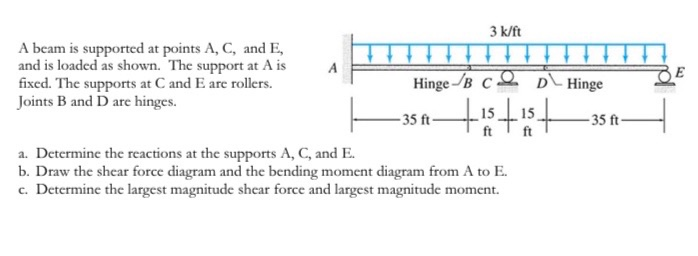3 k/ft A beam is supported at points A, C, and E, and is loaded as shown. The support at A is fixed. The supports at C and E are rollers. Joints B and D are hinges. BE Hinge /B c2 DHinge - 35 ft- - 35 ft- ft ft a. Determine the reactions at the supports A, C, and E. b. Draw the shear force diagram and the bending moment diagram from A to E. c. Determine the largest...

• Required Information For the beam shown, find the reactions at the supports and plot the shear-force...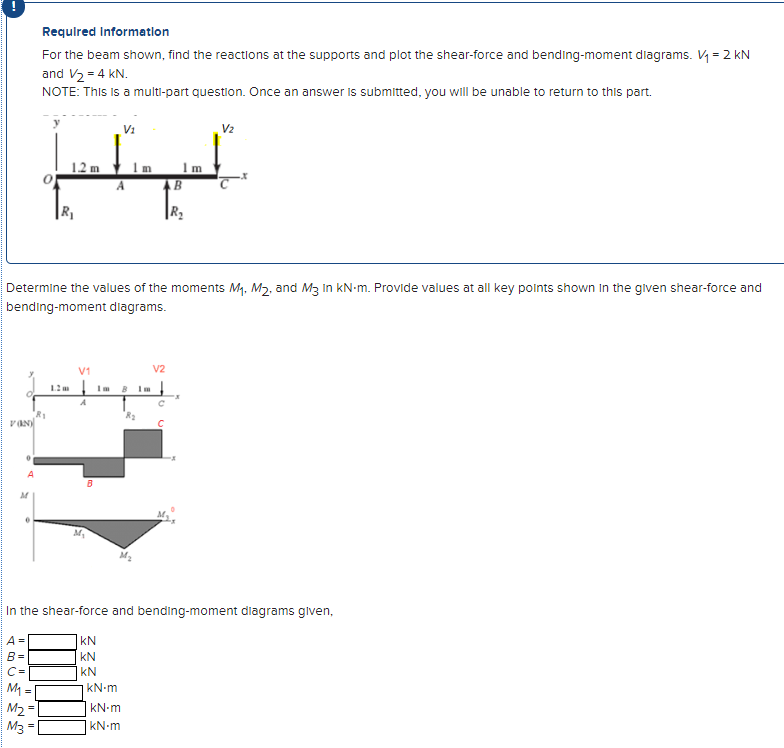Required Information For the beam shown, find the reactions at the supports and plot the shear-force and bending-moment diagrams. V1 = 2 KN and V2 = 4 kN. NOTE: This is a multi-part question. Once an answer is submitted, you will be unable to return to this part. Vi V2 1.2 min А R2 Determine the values of the moments My, M2, and M3 In kNm. Provide values at all key points shown in the given shear-force and bending-moment diagrams....

• Problem 2: For the beams shown below: 1-Calculate the reactions at the supports. 2- Draw the...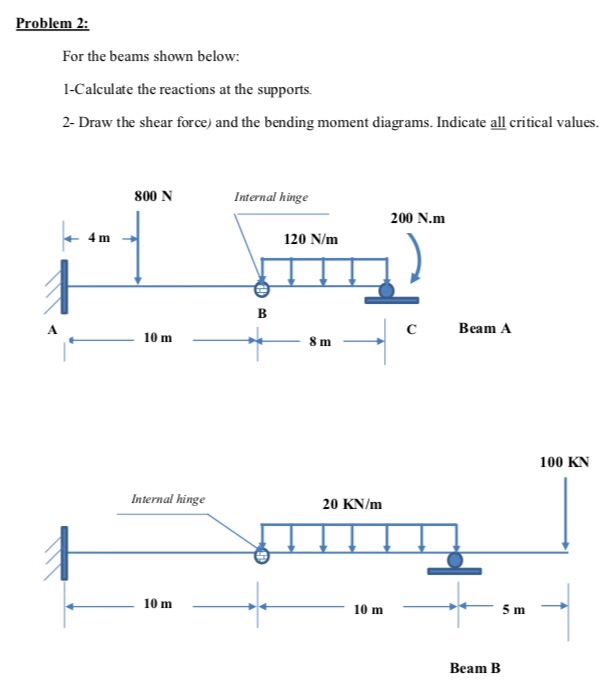Problem 2: For the beams shown below: 1-Calculate the reactions at the supports. 2- Draw the shear force) and the bending moment diagrams. Indicate all critical values. 800 N Internal hinge 200 N.m 4 m 120 N/m Beam A 8 m 100 KN Internal hinge 20 KN/mm 10m ←ㅡㅡㅡ + + 5 m ㅡㅡ 10m ㅡ ㅡ Beam B

• A cantilever beam supports the applied loads and moments as shown. (a) Calculate the support reactions....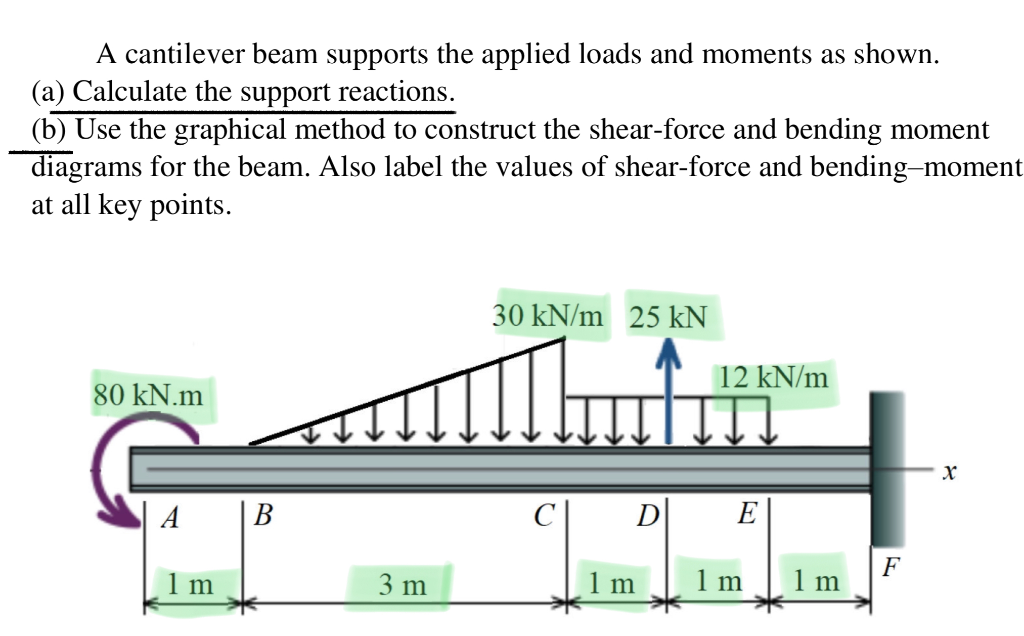A cantilever beam supports the applied loads and moments as shown. (a) Calculate the support reactions. (b) Use the graphical method to construct the shear-force and bending moment diagrams for the beam. Also label the values of shear-force and bending=moment at all key points. 30 kN/m 25 kN 12 kN/m 80 kN.m х A В C D E F 1 m 3 m 1 m 1 m 1 m

• 3. Determine the reactions at the supports shown in the following figure. The beam has a...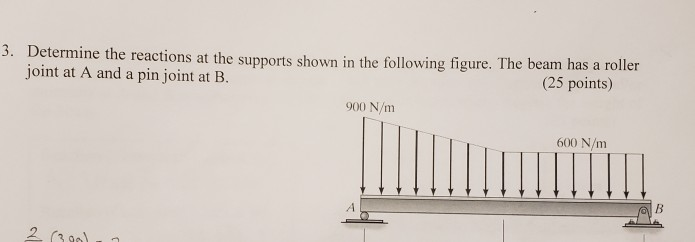3. Determine the reactions at the supports shown in the following figure. The beam has a roller joint at A and a pin joint at B. (25 points) 900 N/m 600 N/m